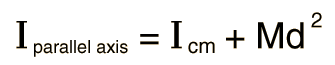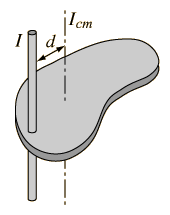# Parallel Axis Theorem

The moment of inertia of any object about an axis through its center of mass is the minimum moment of inertia for an axis in that direction in space. The moment of inertia about any axis parallel to that axis through the center of mass is given byThe expression added to the center of mass moment of inertia will be recognized as the moment of inertia of a point mass - the moment of inertia about a parallel axis is the center of mass moment plus the moment of inertia of the entire object treated as a point mass at the center of mass.Index

Moment of inertia concepts

 HyperPhysics***** Mechanics R Nave
Go Back